# 请问怎么创建一个swap函数

## 假设我定义一个宏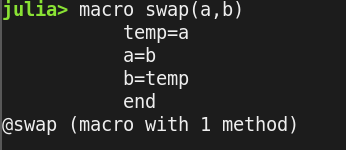## 调用它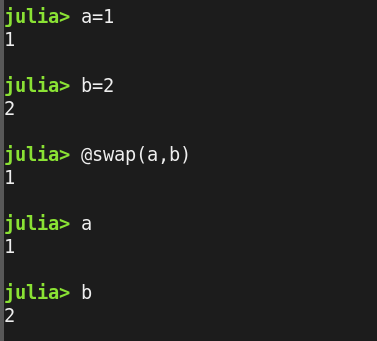## 换一种想法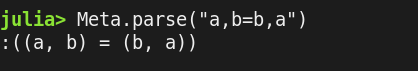## 试着这样定义一个宏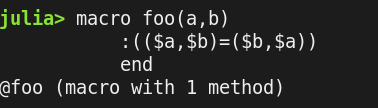## 调用出错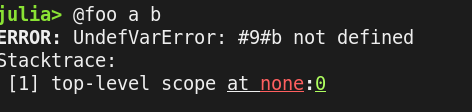## 请问我的宏有没有写错，另外除了a,b=b,a就没有别的方案了吗

``````julia> macro swap(a,b)
:((\$a,\$b)=(\$b,\$a))|>esc
end
@swap (macro with 1 method)

julia> (a,b)=(1,2)
(1, 2)

julia> @swap a b
(2, 1)

julia> (a,b)
(2, 1)
``````

`````````julia
julia> # 代码写在这里

julia> macro swap(a,b)
temp = a
a = b
b = temp
end
@swap (macro with 1 method)

julia>
```
``````

``````julia> # 代码写在这里

julia> macro swap(a,b)
temp = a
a = b
b = temp
end
@swap (macro with 1 method)

julia>
``````

``````macro swap(x, y)
quote
local temp = \$x
\$x = \$y
\$y = temp
end |> esc
end
``````

``````julia> a, b = 1, 2
(1, 2)

julia> a, b
(1, 2)

julia> macro swap(x, y)
quote
local temp = \$x
\$x = \$y
\$y = temp
end |> esc
end
@swap (macro with 1 method)

julia> macroexpand(Main, :(@swap(a,b)))
quote
#= REPL:3 =#
local temp = a
#= REPL:4 =#
a = b
#= REPL:5 =#
b = temp
end

julia> @swap(a, b)
1

julia> a,b
(2, 1)
``````

``````macro swap2(x, y)
quote
\$x,\$y = \$y,\$x
end |> esc
end
``````

``````julia> a, b = 1, 2
(1, 2)

julia> macro swap2(x, y)
quote
\$x,\$y = \$y,\$x
end |> esc
end
@swap2 (macro with 1 method)

julia> macroexpand(Main, :(@swap2(a,b)))
quote
#= REPL:3 =#
(a, b) = (b, a)
end

julia> @swap2(a, b)
(2, 1)

julia> a, b
(2, 1)
``````

thank you both1 个赞